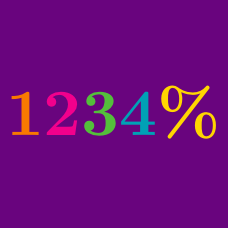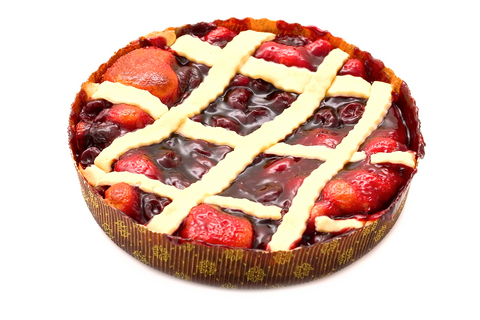Everyday Math

# Percentages: Level 1 Challenges

What is

$1 \% \times \frac{ 100}{10} ?$

$2016 \%\times \frac{2015}{2016}= \, ?$

Katarina is a botanist studying the production of pears by two types of pear trees. She noticed that type A trees produced 20 percent more pears than type B trees did. Based on Katarina's observation, if the type A trees produced 144 pears, how many pears did the type B trees produce?I had a christmas cherry pie sitting in my fridge.
At 1am, my son got hungry and ate $\frac{1}{5}$ of it.
At 2am, my daughter got hungry and ate $\frac{1}{4}$ of what was left.
At 3am, my wife got hungry and ate $\frac{1}{3}$ of what was left.
At 4am, my dog got hungry and ate $\frac{1}{2}$ of what was left.

What percentage of the pie (in %) was left for me in the morning?

Which of these numbers is the largest?

$30\% \text{ of } 10, \hspace{.5cm} 300\% \text{ of } 1, \hspace{.4cm} 10\% \text{ of } 30$

×